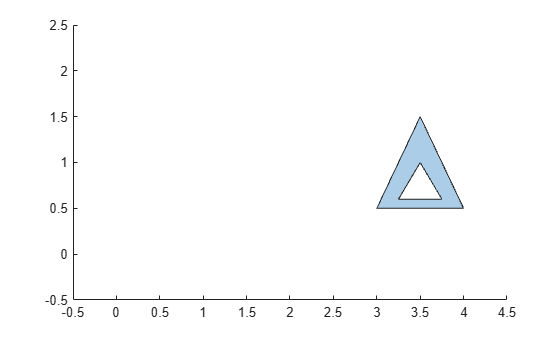regions

Access polyshape regions

Description

example

polyout = regions(polyin) returns a vector of polyshape objects whose elements are the solid regions of the input polyshape.

Examples

collapse all

Compute a polygon with two solid regions. Then, create a vector whose elements are a single region of the polygon.

P = [0 0; 0 2; 2 2; 2 0; NaN NaN; 0.5 0.5; 0.5 1.5; 1.5 1.5; 1.5 0.5; NaN NaN; 3 0.5; 3.5 1.5; 4 0.5; NaN NaN; 3.25 0.6; 3.75 0.6; 3.5 1];
polyin = polyshape(P);
plot(polyin)
xlim([-0.5 4.5])
ylim([-0.5 2.5])polyout = regions(polyin)
polyout =
2x1 polyshape array with properties:

Vertices
NumRegions
NumHoles

plot(polyout(1))
xlim([-0.5 4.5])
ylim([-0.5 2.5])plot(polyout(2))
xlim([-0.5 4.5])
ylim([-0.5 2.5])Input Arguments

collapse all

Input polyshape, specified as a scalar.

Data Types: polyshape